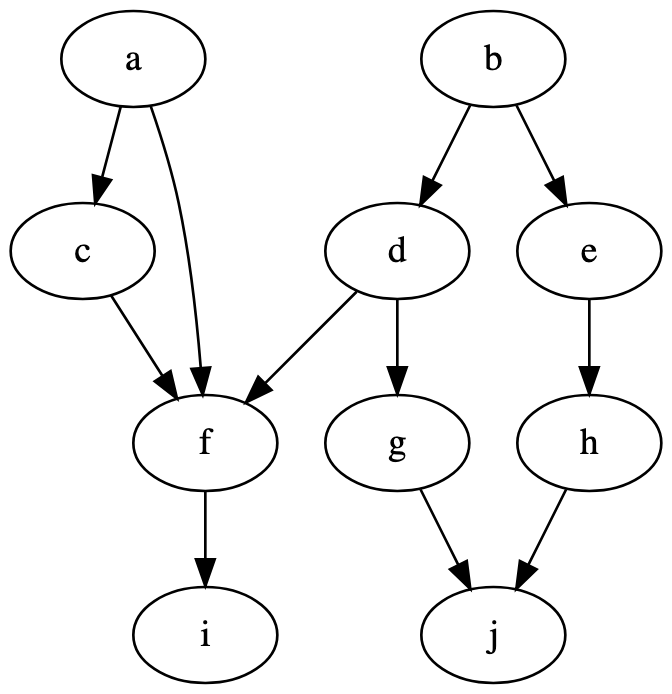Natural Polyglot Machine

# npm

Meet npm Pro: unlimited public & private packages + package-based permissions.Learn more »

## batching-toposort

1.2.0 • Public • Published

# Batching-Toposort

Efficiently sort interdependent tasks into a sequence of concurrently-executable batches.

• `O(t + d)` time complexity (for `t` tasks and `d` dependency relationships)
• `O(t)` space complexity
• Zero package dependencies
• Thoroughly tested (including invariant tests)
• Errors on cyclic graphs

## Motivation

Often one needs to schedule interdependent tasks. In order to determine task order, the classic solution is to use topological sort. However, toposort typically outputs a list of individual tasks, without grouping those that can be executed concurrently. Batching-Toposort takes this additional consideration into account, producing a list of lists of tasks. The outer list is ordered; each inner list is unordered.

## UsageBatching-Toposort expects a directed acyclic graph (DAG) implemented via adjacency list. In other words, construct an object whose keys are dependency IDs, and whose values are lists of dependent IDs.

(If there is demand, Batching-Toposort may one day include a small DAG API and/or DOT support for convenience, but as of now it is the developer's role to construct the graph.)

## Implementation

In short, Batching-Toposort adapts Kahn's Algorithm by inserting each round of root tasks into sublists rather than appending tasks directly to the main output list.

The classic DAG toposort keeps track of each task's in-degree (number of dependencies). As root tasks (those with no dependencies) are added to the output list, their dependents' in-degree counts are decremented. For a task to become a root, all of its dependencies must have been accounted for. The core algorithm is illustrated below in pseudocode (the actual implementation is in `src/index.js`).

``````given G = adjacency list of tasks and dependents (~O(1) lookup):

let N = map from tasks to in-degree counts (~O(1) lookup / update)
let L = [] (empty output list) (~O(1) append)

while R1 is nonempty
append R1 to L
let R2 = [] (empty list for next batch) (~O(1) append)
for each task T in R1
for each dependent D of T (as per G)
decrement in-degree count for D (in N)
if D's in-degree (as per N) is 0
R1 = R2

return L
``````

### Performance

The time complexity is `O(|V| + |E|)` for `V` task vertices and `E` dependency edges.

• The algorithm loops through rounds of roots, and every task is only a root only once, contributing to `O(|V|)` rounds (worst case is a linked list of tasks).
• Each round handles a disjoint set of dependency edges (those rooted in that round's tasks), so the `O(|E|)` handling of all edges is effectively distributed across rounds.
• Other operations, e.g. querying a node's in-degree (average case `O(1)`), are carefully managed to preserve the time complexity.

The space complexity is slightly better at `O(|V|)`.

• The in-degree map size is proportional to the number of vertices `|V|`, but not edges, as those are folded into an integer count during map construction.
• The output by definition contains `|V|` tasks (distributed among as many or fewer lists).
• Again, other operations are controlled to keep space complexity low.

## Keywords

### Install

`npm i batching-toposort`

260

1.2.0

MIT

16.7 kB

7